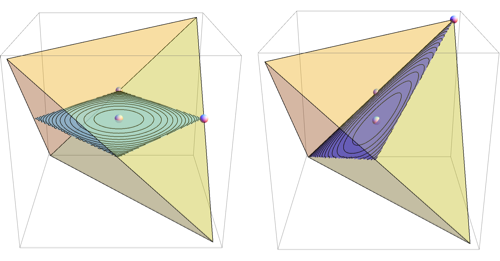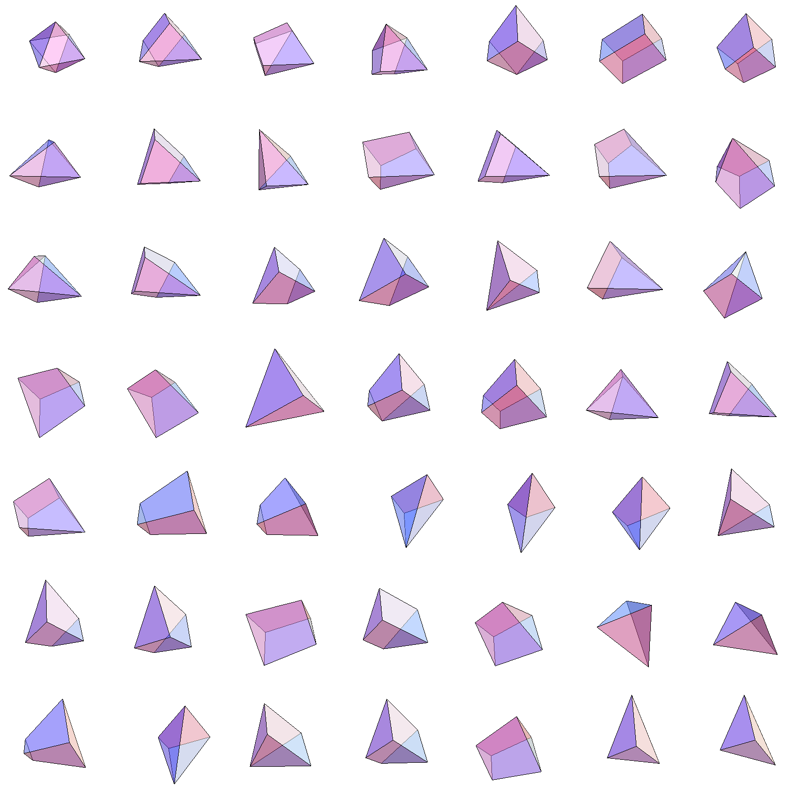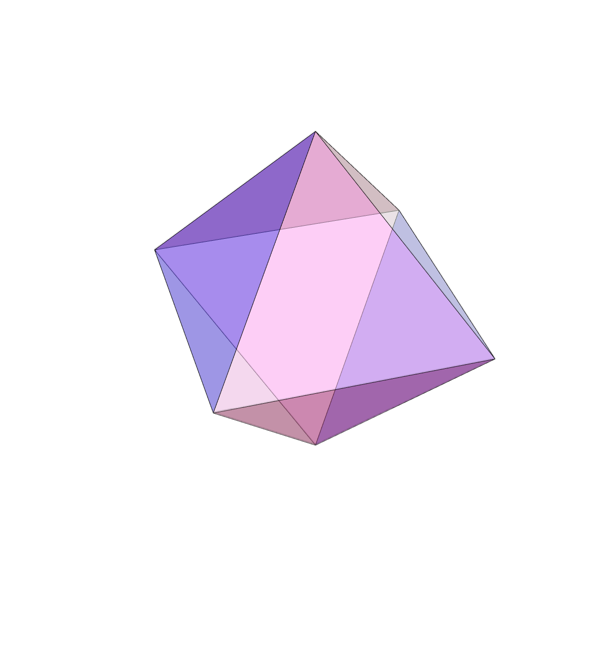## Tuesday, December 21, 2010

### Visualizing 7 dimensional simplex

Suppose we'd like to visualize a set of joint probabilities realizable by distributions of 3 binary variables.

It is a 7 dimensional regular simplex, and we could draw a lower dimensional section of it. There's an infinite number of sections, but there aren't that many *interesting* ones.

As an example, suppose we want to find a good 2 dimensional section of a 3 dimensional regular simplex. It seems reasonable to restrict attention to sections that go through simplex center and two other points, each of which is a vertex or a center of some edge or face. There are just 2 such sections determined up to rotation/reflectionFor 7 dimensional simplex, we could similarly take 3 dimensional sections determined by simplex center and 3 points, each a centroid of some set of vertices. This gives 49 distinct shapes, shown below.Among those, there's just one section that intersects all 8 faces of the simplex and preserves the underlying symmetryAlso known as the regular octahedron.

Implementation details